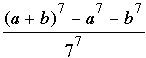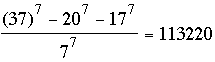Math Central - mathcentral.uregina.caProblem of the MonthCurrentproblem Recent problemswith solutions
 Older problems from 2005/2006 06/07 07/08 08/09 09/10 10/11 11/12

Solution for April 2009

 The Problem:MP85 April 2009 Find integers a and b such that neither 7 nor 18 divides ab(a+b), while 77 does divide (a+b)7-a7-b7.

Correct responses:Correct solutions were sent in this month by

 Bojan Basic (Serbia) Tarik Kaced (France) Ruban Victor Cohen (Argentina) Omran Kouba (Syria) Bernard Collignon (France) Matthew Lim (USA) Allen Druze (USA) Benjamin Louradour (France) Sébastien Dumortier (France) Pavan Manjunath (India) Philippe Fondanaiche (France) John T. Robinson (USA) Cornel Gruian (Romania) Albert Stadler (Switzerland) Edward Doolittle (Regina

The solution:

Our April problem was based on Problem 2 from the 25th International Mathematical Olympiad (Prague, 1984).  The original statement with a solution by Jeremy Kahn can be found in International Mathematical Olympiads 1978-1985, edited by Murray S. Klamkin (Mathematical Association of America, New Mathematical Library #31, 1986).  Most of our correspondents provided a general solution, but Doolittle, Kouba, Lim, and Robinson went further and found necessary and sufficient conditions on the integers a and b for there to exist a solution:

 77 divides (a + b)7 – a7– b7 but neither 7 nor 18 divides ab(a + b) is equivalent to b = 18a + 343k, where a and k are integers for which 7 does not divide a and 9 does not divide ak(a + k); or the same condition holds with the roles of a and b interchanged.

We provide a composite of their similar arguments after first working a few simple examples.  For an example try a = 1; then k = 0 is not allowed (because 9 divides 0), but k = 1 leads to b = 361 and k = –1 leads to b = –325.  For both these examples the quotientis a rather large integer.  For a more tractable example take a = 20 and k = –1; then b = 17 and.

Proof of the claim.  For integers a and b we have

(a + b)7a7b7 = 7ab(a + b)(a2 + ab + b2)2,

so that (a + b)7a7b7 is a multiple of 77 precisely when ab(a + b)(a2 + ab + b2)2 is a multiple of 76.  Moreover, since we are told that 7 does not divide ab(a + b), it follows that 76 divides (a2 + ab + b2)2 and, therefore,

73 divides a2 + ab + b2.

Because 18 × 19 = 342 = 73 – 1, we can factor the quadratic modulo 73 as

a2 + ab + b2 a2 + ab + (1 – 343)b2 ≡ (a + 19b)(a – 18b)
≡ –19(18ab)(a – 18b) ≡ 0 (mod 73).

(For those who are not familiar with the "congruence notation", the display simply tells us that 73 divides evenly into the product –19(18ab)(a – 18b) and, therefore, into the product (18ab)(a – 18b).)  Next, were 7 to divide both 18ab and a – 18b, it would divide their difference 18ab – (a – 18b)= 17(a + b) and, therefore, 7 would necessarily divide a + b, contradicting the assumption that 7 not divide ab(a + b).  We deduce that 73 divides either 18ab or a – 18b, but not both.  In the former case, should 73 divide 18ab then, for any integer a there exists an integer k for which b = 18a + 343k, whence the condition that 7 not divide ab(a + b) = a(18a + 343k)(19a + 343k) ≡ 6a3 (mod 7) reduces to 7 does not divide a.
It remains to consider the further stipulation that 18 not divide ab(a + b), or (because that product is necessarily even) more simply, that 9 not divide ab(a + b).  Again letting b = 18a + 343k, we have

ab(a + b) = a(18a + 343k)(a + 18a + 343k) ≡ ak(a + k)  (mod 9),

and we deduce that 18 divides ab(a + b) if and only if 9 divides ak(a + k).  To summarize, we have determined that for the integers a and b to be solutions to our problem we must have either

b = 18a + 343k for integers a and k such that 7 does not divide a and
9 does not divide ak(a + k),
or
a = 18b + 343k for integers b and k such that 7 does not divide b and
9 does not divide bk(b + k).

Because each step was reversible, it is easy to check that our necessary conditions are also sufficient.

Most of the submitted solutions did not factor a2 + ab + b2, although they did determine (as we saw above) that it must be a multiple of 73.  Allen Druze observed that one can easily find a solution, such as a = 3, b = 6, to the much simpler problem of finding a and b such that 7 divides a2 + ab + b2.  Other members in this family of solutions have the form a = 3 + 7k, b = 6 + 7n, and one quickly sees a pattern that leads to finding integer pairs such as (a, b) = (–32, –29) for which 49 divides a2 + ab + b2; one then uses –32 + 49k and –29 + 49n (with new k and n) to obtain finally a pair such as (17, 20) for which 343 divides 172 + 17·20 + 202.  Bojan Basic used a more systematic version of this approach based on Hensel's Lemma; he provided the Wikipedia reference
http://en.wikipedia.org/wiki/Hensel%27s_lemma .
He observed, however, that it is much easier to type a one-line command in some computer algebra system such as Mathematica, and let it find the solutions.
Other successful approaches involved letting the variable x represent the unknown a and treating b as a constant, then solving the congruence x2 + bx + b2 ≡ 0 by your favorite method, or solving the related quadratic equation

x2 + bx + b2 = 343k

for small integer values of k.  We know (from our detailed solution above) that such an approach will find infinitely many solutions because for any fixed b there will be many appropriate values of k.  For example, if we let b = 17 and k = 3, then x = 20 and–37, which we interpret to mean that when b = 17 we get possible solutions when a = 20 and a = –37.  It remains only to check that neither 7 nor 18 divides the resulting product
ab(a + b).

 Math Central is supported by the University of Regina and the http://mathcentral.uregina.ca/QQ/database/QQ.09.06/joe3.html.about math central :: site map :: links :: notre site français